APPLIED INDUSTRIAL CONTROL SOLUTIONS LLC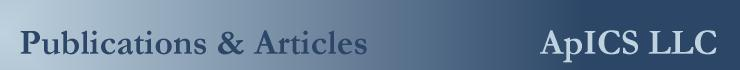PAPER No. 8

Estimating Web Strain, Modulus of Elasticity and Loss Torque Using an Extended Kalman Filter

1999 International Web Handling Conference

Brian Thomas Boulter
Applied Industrial Control Solutions LLC
4597 E Sprague Rd.
Independence, OH, USA 44131

Abstract: A set of sub-optimal Extended Kalman Filters (EKF's) are described. The filters are used to estimate web tension, modulus of elasticity, and loss-torque for all sections in a small web processing line located at Rockwell Automation's Euclid Research Facility. Conditions necessary for observability, and estimate convergence are discussed

I. Introduction

A. Nomenclature

 I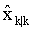State estimate vector at the kth epoch given the predicted state vector from the previous epoch.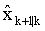Noise-free predicted state vector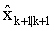Predicted state vector (with residuals)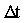Discrete sample time [sec]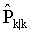Updated probability density matrix from the previous epoch.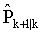Linearized Predicted probability density matrix, given the update from the previous epoch.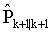Updated probability density matrix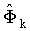State Transition Matrix at the kthepoch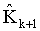Updated Kalman filter gain matrix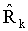Sensor noise covariance matrix at the kth epoch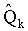Process noise covariance matrix at the kth epoch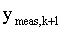Measured states at the kth epoch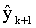Predicted outputs, given the noise-free predicted states. I The identity matrix e Web strain [engineering units of choice] t Motor torque [engineering units of choice] tL loss-torque [same units as t] Ai ith web cross sectional area [engineering units of choice] Ti ith tenion [units of force]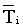ith normalized tension Ei ith modulus of elasticity [units of force per units of A]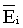ith normalized modulus of elasticity [1=EREF] zi ith strain factor 1/(1+ e) wi ith roll rotational speed [rad/sec]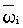ith normalized rotational speed [1=wb,i] wb,i ith motor base speed [rad/sec] Li ith length [engineering units of choice] Ri ith roll radius [engineering units of choice] GRi ith gear ratio wj,i jth state in the ith zone, zero-mean white noise terms.

B. Problem Definition

The EKF is often used in the aerospace industry to estimate states and/or important system parameters in noisy systems. Why do we need to concern ourselves with this kind of technology in the drive systems business?

There are many sources of uncertainty in a web processing system, for example, tension measurements can be corrupted by incorrect calibration of the load cell or strain gauge. The same can be said for the uncertainty associated with calculation of roll diameter as a function of an estimated surface speed and its ratio to the winder motor speed in ratio detector algorithms. Time varying frictional losses add uncertainty to friction compensation algorithms as do slight changes in material density to inertia compensation algorithms. The estimation of motor torque from a current reference in DC or torque reference in AC as compared to the actual motor shaft torque introduces another source of uncertainty. In the paper and film industries, time-varying modulus of elasticity adds another source of uncertainty. A Kalman filter that is designed to estimate states based on a known model of the uncertainty in the measured states is desirable.

A Luenberger observer, can be implemented [5,6] to estimate system states, but such an implementation is not robust in the presence of noise. This is especially true for metals applications. Because of the high gain in the relationship between stress and strain (Young’s modulus) in metals applications, noise in the speed feedback can result in large errors in the strain estimation. A Kalman filter that is designed to provide reliable estimates of important system parameters in the presence of well modeled system noise would be most useful. An additional benefit provided by such a filter, is that it could estimate unmeasured parameters, such as web strain, and loss-torque.

The Kalman filter can provide the user with a measure of how "good" the estimate is. This measure, the covariance of the estimate, is most accurate in systems where the uncertainty is Gaussian, and all process and sensor noise is band-limited and white. In systems where there are cyclical disturbances (e.g. machine resonances) and the resulting noise is pink at certain frequencies, a Kalman filter can be designed to filter the cyclic noise and calculate un-biased parameter and state estimates , however, the design of the Kalman filter in these instances is significantly more complex, for this reason pink noise sources will not be considered in this presentation.

The usual drawback to the implementation of any optimal EKF is the need to heuristically derive optimum noise covariance matrices Q and R. Another is the trade-off between the need for computing power, and the need for filter bandwidth (i.e. update time). Most implementations usually result in such long update times that the Filter estimates are of little practical use in real time systems.

Figure 7. Shows the optimal EKF implementation for the Euclid lab-line system. In this system there are 22 system states, and 12 system inputs. To execute the EKF algorithm with this many states on a 7010 Automax ® processor would take approximately 20 [sec] per update. Clearly, with such an update time, the tension estimates could not be used to close a sufficiently responsive tension loop.

However, if a set of sub-optimal filters are designed, and linked with estimated parameters, as shown in Figure 8, the resulting sub-optimal filter computational requirements are significantly reduced. In this case the most number of estimated states is 3 and the most number of inputs is 2. The resulting matrices are at most 3x3, and all matrix computations can be accomplished with simple routines. The EKF algorithm can now be executed in about 15 [msec] using a 7010 Automax processor. Given that the scan-time of a standard Rockwell Automation tension loop is 22 [msec], tension estimates, for example, can be used to close a tension loop.

Having overcome the implementation hurdle, the process of exercising the EKF on the Euclid lab-line revealed that the estimated web strain, loss-torque, and web modulus of elasticity states, in those sections where the tension feedback was not made available to the filter, would not converge. A dual state analysis performed by Angus Andrews at the Rockwell Science Center  provided a proof that tension feedback was required if these states were to be estimated. The conclusions drawn in the proof were extensible to Luenberger observers, implying that any observer or state estimator of web strain, loss-torque, or modulus of elasticity was not feasable if it is implemented without tension feedback. This was an important conclusion given that the impetus for the study was to investigate the possibility of developing tension sensor-less web process lines.

C. Paper Overview

The first section contains a brief description of the EKF algorithm. The second section describes the non-linear process model used in the final sub-optimal Kalman filter implemented on the Euclid lab-line. The third section describes the coding of the EKF for use with the Automax distributed control system, and results from the integration of the filter onto the Euclid lab-line. Conditions necessary for observability and estimate convergence, along with conclusions are presented in the fourth section.

II. The Extended Kalman FIlter Algorithm

The EKF algorithm described by Figures 4 and 5, and derived in numerous texts on the subject ([1,2,3,4]) is summarized below. The noise statistics are modeled as follows: Let Q and R be the covariances of zero-mean Gaussian process noise ‘w’ and the measurement noise ‘v’ respectively: Then:

State prediction equations: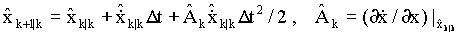Covariance prediction equations: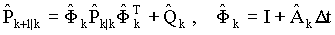Kalman gain equations for each sub-optimal section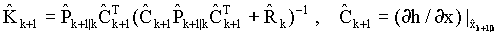Covariance update equations: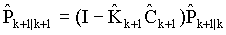State update equations: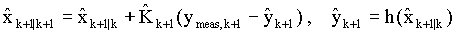III. System Modeling and Filter Implementation

The Euclid lab-line (Figure 1.) is a four section web processing line designed for the evaluation of web handling control strategies. For this study the web material used in the process was Kraft paper. A one-line diagram of the lab-line is shown in Figure 2. A simplified one-line diagram is shown in Figure 3.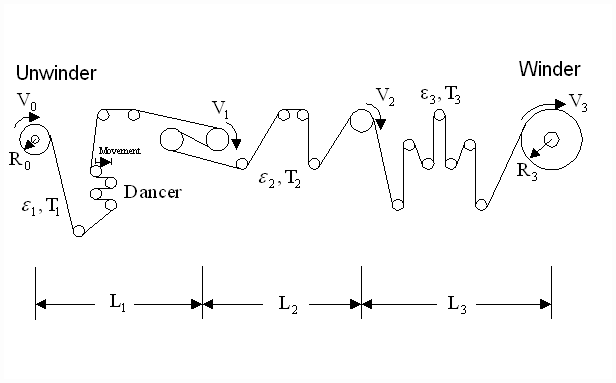Figure 1. Euclid Lab-Line FacilityFigure 2. Lab-Line Physical Model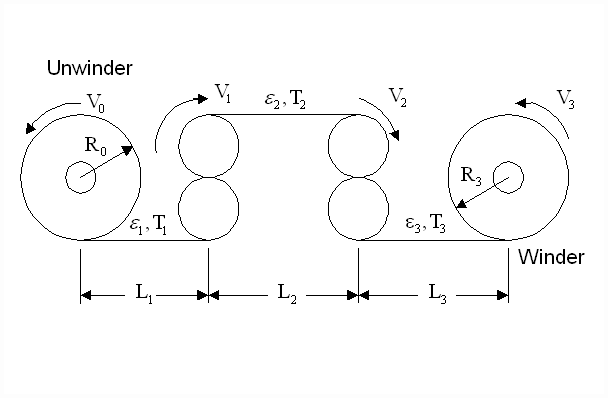Figure 3. Simplified Lab-Line Model

A. State Variable Designations

The EKF algorithm described above (Figures 4, and 5), requires the a-priori designation of all system state variables, inputs, outputs, measured state variables, estimated state variables, process noise sources, and sensor noise sources. These designations are made based on a satisfactorily complete model of the process.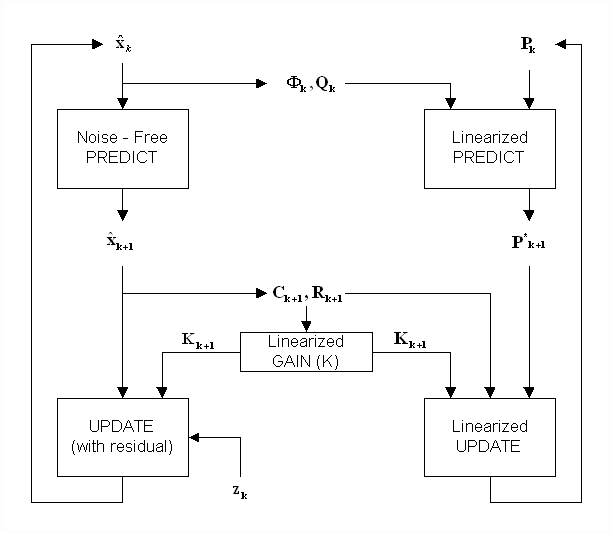Figure 4. Algorithm Structure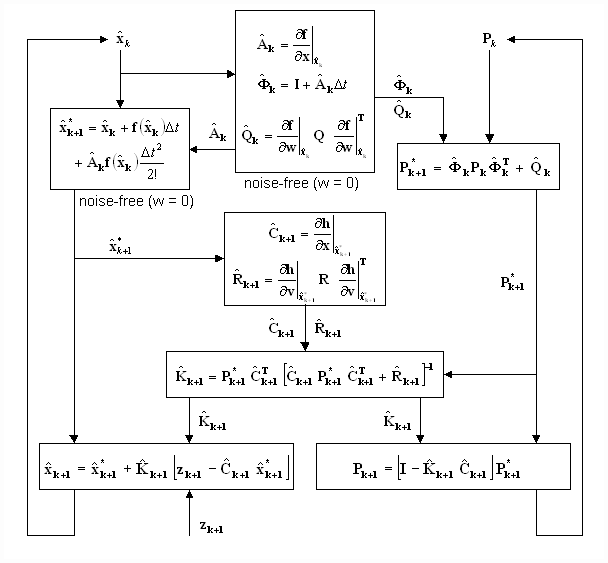Figure 5. Extended Kalman Filter Algorithm

Unwinder + Downstream Web (With Dancer Disabled as a Torque Regulator)

Measured states:
Roll (angular) speed
Roll diameter (from ratio detector)
Roll torque: t = f(current & field strength)

Estimated states:
Web strain factor: z = 1/(1+e ), where e = strain
Roll loss-torque: t L
Web modulus of elasticity: E

Tensions:
Input (wound-on): assumed zero
Output (downstream): calculate, T = AEe = AE(1/z –1)

S-Wrap Bridle + Downstream Web

Measured states
Roll (angular) speed
Roll torque: t = f(current & field strength)

Estimated states
Web strain factor: z = 1/(1+e ), where e = strain
Roll loss-torque: t L
Web modulus of elasticity: E

Tensions
Input: from upstream subsystem
Output (downstream): calculate, T = AEe = AE(1/z –1)

Pull-Roll + Downstream Web

Measured states:
Roll (angular) speed
Roll torque: t = f(current & field strength)

Estimated states:
Web strain factor: z = 1/(1+e ), where e = strain
Roll loss-torque: t L
Web modulus of elasticity: E

Tensions:
Input: from upstream subsystem
Output (downstream): calculate, T = AEe = AE(1/z –1)

Rewinder

Measured states:
Roll (angular) speed
Roll diameter (from ratio detector)
Roll torque: t = f(current & field strength)

Estimated state:
Roll loss-torque: t L

Tensions:
Input: from upstream subsystem
Output (downstream, wound-in): assumed zero

B. First Principal Equations

The following first principal equations were used to describe each section of the simplified system (Figures 3 and 6) in each of the sub-optimal EKF’s (Figure 8). Note: for the sake of brevity, the Unwinder was configured as a torque regulator, and the estimator for the dancer position was not implemented. Note: the choice of units must be made appropriately so that the equation dimensions are correct.

State Equations

 Upstream: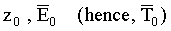Downstream: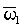Motor speed: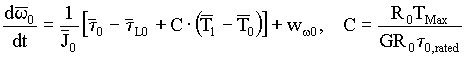Motor torque: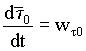Loss-torque: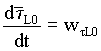Strain factor: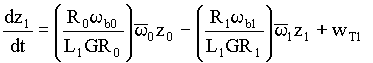Modulus of elasticity: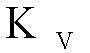Where: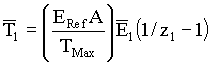Note: The wi terms represent the zero-mean noise terms (covariance = Qi ).

The subsystem model equations for the Unwinder and Rewinder are slightly different than those shown above for constant diameter rolls. First, an additional state equation is necessary in both the Unwinder and Rewinder to estimate the varying roll diameter; a measurement of the diameter is available in each case (from a ratio detector). And second, the Rewinder does not have a downstream web, so the state equation for strain factor is eliminated. The derivation of these equations is left up to the reader.

C. Lab-Line Implementation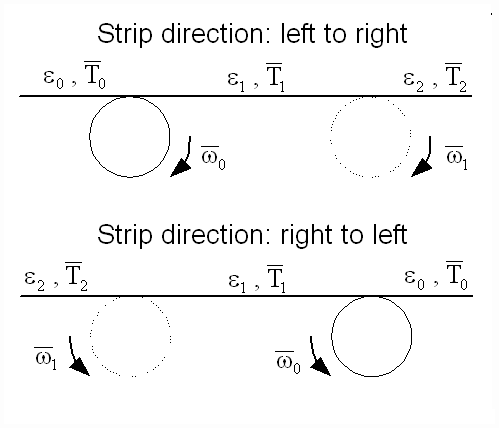Figure 6. Subsystem Model Structure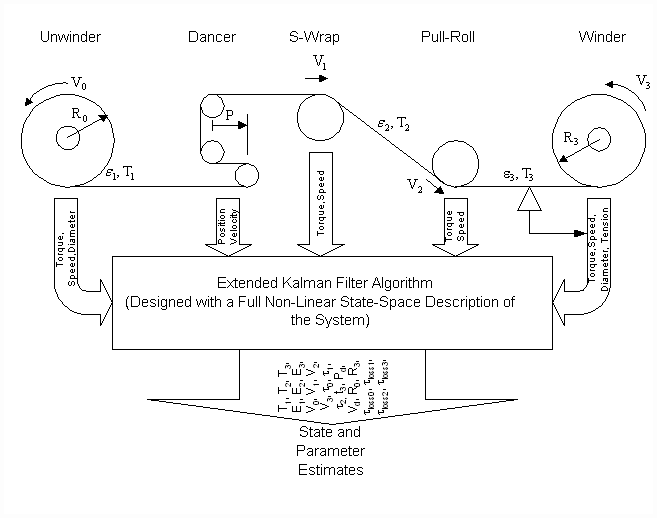Figure 7. Optimal Kalman Filter Design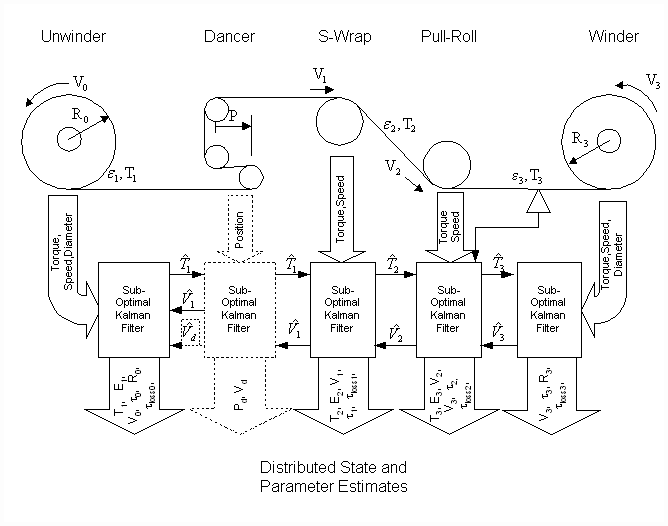Figure 8. Sub-Optimal Kalman Filter Design

Each subsystem model was implemented as one roll plus downstream web, as illustrated in Figure 6. The subscript 0 represents upstream and the subscript 1 represents downstream. The implementation was structured with one AutoMax 7010 processor card per sub-optimal filter. Programming was done using AutoMax enhanced BASIC equations. Software switches were included for correctly re-assigning variables when reversing line speed direction. The various measured state variables from each subsystem were observed using the Sigma ® data system during line operation in the forward and reverse directions. Data was extracted and plotted using the Mathworks Corp. MATLAB ® data presentation algorithms.

IV. Observations

Estimated states for the line between the Pull-roll and the Rewinder were the most well-behaved. The estimated modulus of elasticity for the web section between the two rolls tended to settle at a steady-state value that was reasonably close to the expected value. The reason for these well-behaved results was later proven to be due to the presence of a tension measurement in this zone.

Estimated states for other regions of the line tended to deviate from expected values, similar to the deviations noted during MATLAB simulations of the Lab-line. In particular, the modulus of elasticity values for the two other web sections – i.e., the S-wrap to Pull-roll section and the Unwinder to S-wrap section, appeared to diverge either above or below their expected values, depending on the covariance values selected for the Q-matrix and R-matrix elements. This indicated that the modulus of elasticity and loss-torque are not observable, unless there is a measurement of tension available.

The results presented below for the S-Wrap section loss-torque estimation illustrate these points. The measured states are angular speed, w 0 , and motor torque, t 0. The estimated states also included: strain factor, z1 , and a calculated modulus of elasticity, E1. For the diagonal Q matrix covariances given in Table 1, the results are shown in Figures 9 through 11:

 State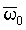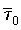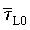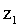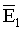Q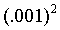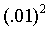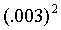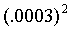RTable 1.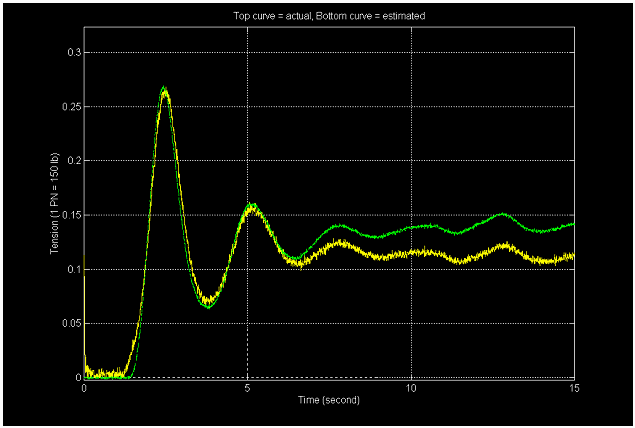Figure 9. Actual tension (green) versus Kalman filter estimated tension (yellow)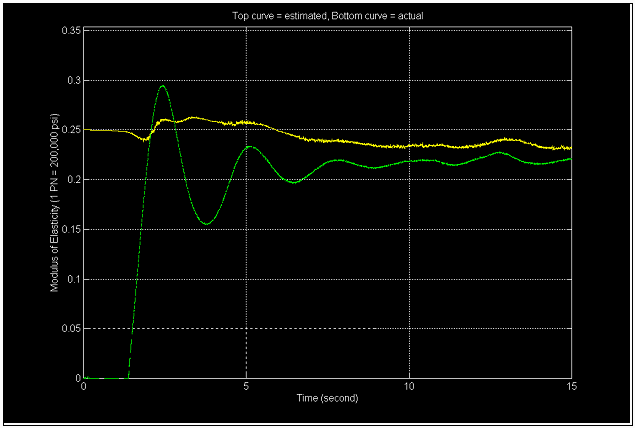Figure 10. Calculated modulus of elasticity (green) versus Kalman filter estimate (yellow)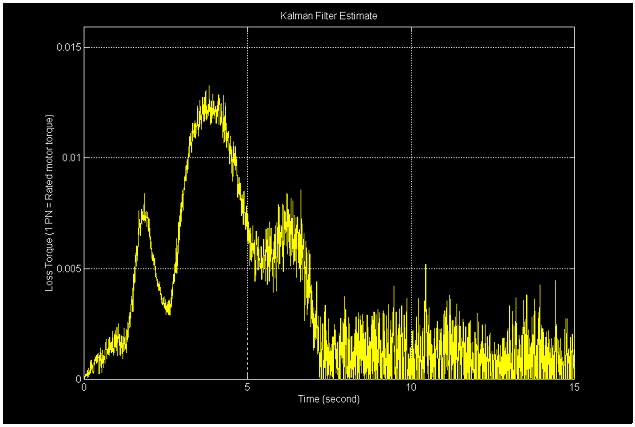Figure 11. Loss-torque estimated by Kalman filter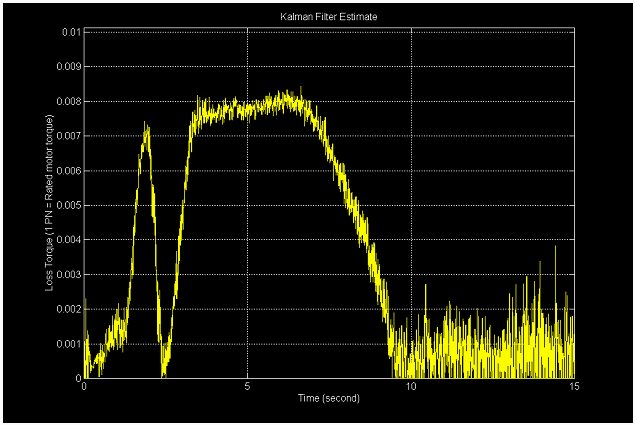Figure 12. Estimated loss-torque for cross coupling terms Q(1,3) = Q(3,1) = (0.006)^2.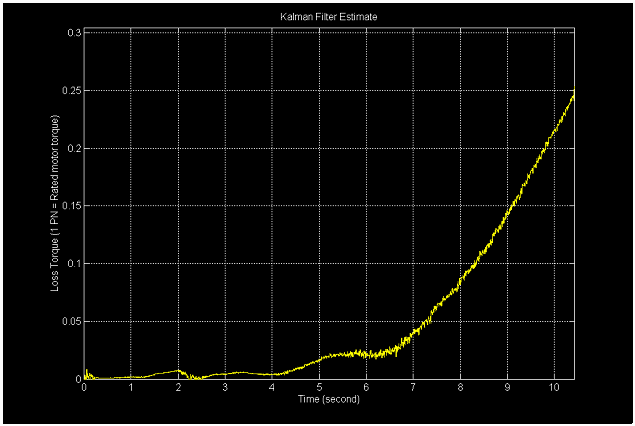Figure 13. Estimated loss-torque for cross coupling terms Q(1,3) = Q(3,1) = (0.01)^2

Increasing the process covariance for the modulus of elasticity from (.003)2 to (.01)2 caused the estimated value of E1 to diverge above the actual. Decreasing the process covariance for E1 from (.003)2 to (.001)2 caused the estimated modulus of elasticity to diverge below the actual value. Similar heuristic strategies were employed for optimally setting the co-variances of all other state variables. The effect of off-diagonal values in the Q matrix were then investigated.

The most likely off-diagonal terms to be present in the above example were assumed to be the ones linking loss-torque and roll angular speed; i.e., most likely, t L » Bv w . If roll angular speed is state 1 and loss-torque is state 3, then the cross-coupled process covariance becomes the matrix elements Q(1,3) = Q(3,1). Estimation of loss-torque appeared to approach a steady-state value only when these cross-coupling terms were at or near a critical value. Much effort was expended in investigating and identifying optimal settings for all diagonal and cross-coupling covariances. For example, decreasing the cross-coupled process covariance, loss-torque to speed, Q(1,3) = Q(3,1) from (.008)2 to (.006)2 resulted in the loss-torque estimate shown in Figure 12. Whereas increasing the cross-coupled process covariance, loss-torque to speed, Q(1,3) = Q(3,1) from (.008)2 to (.01)2 resulted in the unbounded loss-torque estimate shown in Figure 13. Similar observations were made while setting other estimated state process and sensor noise covariance values.

It is believed that the estimation of Young’s modulus would be significantly improved if a model relating stress to strain for the material in the web process line was implemented in the above state equations. The model used in the Kalman filter on the lab-line was composed of only a noise model. Several models were investigated, they were obtained heuristically with measurements of web stretch as a function of tension, however, slight changes in humidity rendered them inaccurate. The stress strain relationship of metals appears to be much better understood and modeled. It is believed that the Kalman filter will perform significantly better in metal processes where these models can be implemented. The author noticed that, in the area of paper, film, and plastics, there is a lack of published material on this subject.

V. Conclusions

The author corresponded with Angus Andrews at the Rockwell Science Center for assistance when it was observed that the state estimates of tension, strain factor, web modulus of elasticity, and loss-torque would not converge in those sections where a tension measurement was not fed back into the EKF. A dual state analysis of the system was performed  and a comparison of optimal and sub-optimal filters revealed that the above states were only observable if a measurement of at least one of the above mentioned states was made available to each the sub-optimal filters. Tension is the only state that can be practically measured. This conclusion implied that the implementation of a tension sensor-less system is not feasible. However, important states, such as web strain, or loss-torque, can be estimated if the tension feedback is made available, and an EKF is used to estimate these states, even in the presence of significant process and sensor noise.

The structure and results of the dual state analysis will be the subject of another paper on this topic. However, by inspection of the equations describing strain factor, tension, loss-torque (i.e. speed), it is clear that the above states exhibit dependence. For example, if the estimate of web tension is lower than the actual, the estimate of loss-torque must be higher than the actual, this is because the estimate of shaft torque is accompanied by a measurement. It can be considered known with a degree of certainty. At a steady state speed, the torque on the shaft minus loss-torque, will produce strain in the material. What is lost in one estimate must be made up in the other estimate.

VI. Acknowledgements

The author would like to express his gratitude to the following people who, provided assistance in the completion of this study. Dr. Alan S. Wilsky, and Dr. Robert R. Tenney of the Massachusetts Institute of Technology for their "Fundamentals of Detection, Parameter Estimation, and Kalman Filtering" summer course . Dr. Angus Andrews of the Rockwell Science Center for his insights into Kalman Filtering and dual state analysis techniques , . Harold W. Fox of ApICS LLC for his help in analyzing the theory, his work in coding the non-optimal Kalman Filters into Automax Extended Basic, and his help in undertaking the testing. We would also like to thank Rockwell Automation for their permission to use the Euclid Lab Line for testing the algorithms.

References

 Kalman, R. E., "A New Approach to Linear Filtering and Prediction Problems", Transactions of the ASME, J. Basic Engineering, Vol. 82 pp 34-45, 1960.

 Willsky, Alan S., Tenney, Robert R., "Fundamentals of Detection, Parameter Estimation and Kalman Filtering", M.I.T. Professional Institute Course Materials, 1997.

 Gardner, William A., "Introduction to Random Processes With Applications to Signals and Systems", McGraw Hill ISBN 0-07-022855-8 1989.

 Kailith, T., "Linear Systems", Englewood Cliffs, N.J.: Prentice-Hall. 1980.

 Wolferman, W., "Tension Control of Webs - A Review of the Problems and Solutions in the Present and Future", Tab 15, Proceedings of the 3rd IWHC International Web Handling Conference, Oklahoma State University June 1995.

 Wolferman, W., "Sensorless Tension Control of Webs", Tab 23, Proceedings of the 4th IWHC International Web Handling Conference, Oklahoma State University June 1997.

 Andrews, Angus, "Appendices on Reducing Tension Sensor Requirements in Web Processing", Unpublished, Internal Rockwell Automation engineering document, 1997.

 Andrews, Angus, "Dual State Analysis of Web Processing Line Models", Unpublished, Internal Rockwell Automation engineering document, 1998.